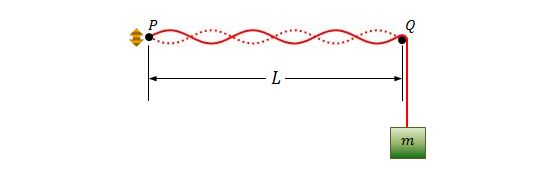Classical Mechanics

# Waves - Problem SolvingYou are watching a marching band practice outdoors. Near you are two trumpet players, one marching directly towards you and one away from you at the same speed. Each trumpet player is playing an A (440 Hz) and you hear a beat between the two sounds at a frequency of 2 Hz. How fast are the trumpet players marching in m/s?

Details and assumptions

• The speed of sound is $340~\mbox{m/s}$.
• The trumpet players aren't moving very fast.

A wire with mass $100\text{ g}$ and length $10.00\text{ m}$ is held on the two points $P$ and $Q$ under a tension of $240\text{ N}.$ Pulse $1$ is sent along the wire from $P$ at time $t=0,$ and pulse $2$ is sent along the wire from $Q$ at time $t=35.0\text{ ms}.$ Approximately, at what distance $x$ from $P$ do the pulses begin to meet?A string, which is fixed at point $P,$ is stretched by a block with mass $m$ at point $Q,$ as shown in the above figure. Point $P$ is generating a sinusoidal wave but the amplitude of $P$ is small enough to be considered as a node. The distance between $P$ and $Q$ is $L=1.50\text{ m},$ and the linear density of the string is $\mu=1.60\text{ g/m}.$ If the oscillating frequency of $P$ is $f=130\text{ Hz}$ and is setting up sixth harmonic on the string, what is the approximate mass $m$ of the block?

Assumptions and Details

• The gravitational acceleration is $g=9.80\text{ m/s}^2.$

If the linear densities of the heaviest and lightest steel wires of a certain piano are $3.1 \text{ g/m}$ and $0.26\text{ g/m},$ respectively, what is the approximate ratio of the diameter of the two wires?

What is the approximate speed of a transverse wave in a rope of length $1.75\text{ m}$ and mass $60.0\text{ g}$ under a tension of $700\text{ N}?$

×